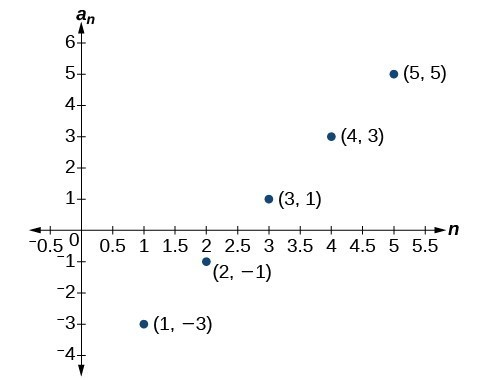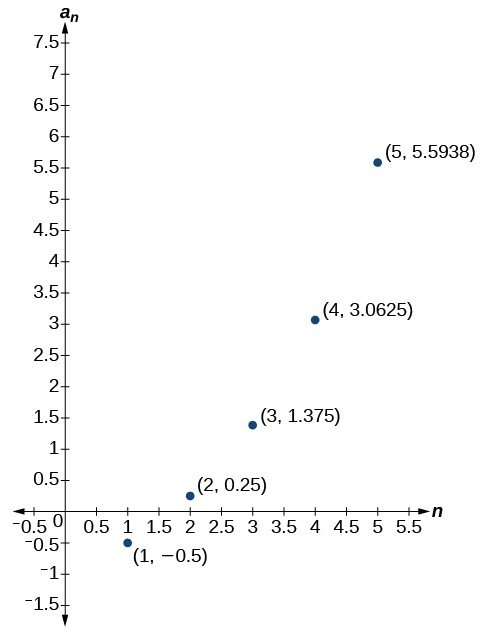## Section Exercises

1. What is a geometric sequence?

2. How is the common ratio of a geometric sequence found?

3. What is the procedure for determining whether a sequence is geometric?

4. What is the difference between an arithmetic sequence and a geometric sequence?

5. Describe how exponential functions and geometric sequences are similar. How are they different?

For the following exercises, find the common ratio for the geometric sequence.

6. $1,3,9,27,81,..$.

7. $-0.125,0.25,-0.5,1,-2,..$.

8. $-2,-\frac{1}{2},-\frac{1}{8},-\frac{1}{32},-\frac{1}{128},..$.

For the following exercises, determine whether the sequence is geometric. If so, find the common ratio.

9. $-6,-12,-24,-48,-96,..$.

10. $5,5.2,5.4,5.6,5.8,..$.

11. $-1,\frac{1}{2},-\frac{1}{4},\frac{1}{8},-\frac{1}{16},..$.

12. $6,8,11,15,20,..$.

13. $0.8,4,20,100,500,..$.

For the following exercises, write the first five terms of the geometric sequence, given the first term and common ratio.

14. $\begin{array}{cc}{a}_{1}=8,& r=0.3\end{array}$

15. $\begin{array}{cc}{a}_{1}=5,& r=\frac{1}{5}\end{array}$

For the following exercises, write the first five terms of the geometric sequence, given any two terms.

16. $\begin{array}{cc}{a}_{7}=64,& {a}_{10}\end{array}=512$

17. $\begin{array}{cc}{a}_{6}=25,& {a}_{8}\end{array}=6.25$

For the following exercises, find the specified term for the geometric sequence, given the first term and common ratio.

18. The first term is $2$, and the common ratio is $3$. Find the 5th term.

19. The first term is 16 and the common ratio is $-\frac{1}{3}$. Find the 4th term.

For the following exercises, find the specified term for the geometric sequence, given the first four terms.

20. ${a}_{n}=\left\{-1,2,-4,8,…\right\}$. Find ${a}_{12}$.

21. ${a}_{n}=\left\{-2,\frac{2}{3},-\frac{2}{9},\frac{2}{27},…\right\}$. Find ${a}_{7}$.

For the following exercises, write the first five terms of the geometric sequence.

22. $\begin{array}{cc}{a}_{1}=-486,& {a}_{n}=-\frac{1}{3}\end{array}{a}_{n - 1}$

23. $\begin{array}{cc}{a}_{1}=7,& {a}_{n}=0.2{a}_{n - 1}\end{array}$

For the following exercises, write a recursive formula for each geometric sequence.

24. ${a}_{n}=\left\{-1,5,-25,125,…\right\}$

25. ${a}_{n}=\left\{-32,-16,-8,-4,…\right\}$

26. ${a}_{n}=\left\{14,56,224,896,…\right\}$

27. ${a}_{n}=\left\{10,-3,0.9,-0.27,…\right\}$

28. ${a}_{n}=\left\{0.61,1.83,5.49,16.47,…\right\}$

29. ${a}_{n}=\left\{\frac{3}{5},\frac{1}{10},\frac{1}{60},\frac{1}{360},…\right\}$

30. ${a}_{n}=\left\{-2,\frac{4}{3},-\frac{8}{9},\frac{16}{27},…\right\}$

31. ${a}_{n}=\left\{\frac{1}{512},-\frac{1}{128},\frac{1}{32},-\frac{1}{8},…\right\}$

For the following exercises, write the first five terms of the geometric sequence.

32. ${a}_{n}=-4\cdot {5}^{n - 1}$

33. ${a}_{n}=12\cdot {\left(-\frac{1}{2}\right)}^{n - 1}$

For the following exercises, write an explicit formula for each geometric sequence.

34. ${a}_{n}=\left\{-2,-4,-8,-16,…\right\}$

35. ${a}_{n}=\left\{1,3,9,27,…\right\}$

36. ${a}_{n}=\left\{-4,-12,-36,-108,…\right\}$

37. ${a}_{n}=\left\{0.8,-4,20,-100,…\right\}$

38. ${a}_{n}=\left\{-1.25,-5,-20,-80,…\right\}$

39. ${a}_{n}=\left\{-1,-\frac{4}{5},-\frac{16}{25},-\frac{64}{125},…\right\}$

40. ${a}_{n}=\left\{2,\frac{1}{3},\frac{1}{18},\frac{1}{108},…\right\}$

41. ${a}_{n}=\left\{3,-1,\frac{1}{3},-\frac{1}{9},…\right\}$

For the following exercises, find the specified term for the geometric sequence given.

42. Let ${a}_{1}=4$, ${a}_{n}=-3{a}_{n - 1}$. Find ${a}_{8}$.

43. Let ${a}_{n}=-{\left(-\frac{1}{3}\right)}^{n - 1}$. Find ${a}_{12}$.

For the following exercises, find the number of terms in the given finite geometric sequence.

44. ${a}_{n}=\left\{-1,3,-9,…,2187\right\}$

45. ${a}_{n}=\left\{2,1,\frac{1}{2},…,\frac{1}{1024}\right\}$

For the following exercises, determine whether the graph shown represents a geometric sequence.

46.47.For the following exercises, use the information provided to graph the first five terms of the geometric sequence.

48. $\begin{array}{cc}{a}_{1}=1,& r=\frac{1}{2}\end{array}$

49. $\begin{array}{cc}{a}_{1}=3,& {a}_{n}=2{a}_{n - 1}\end{array}$

50. ${a}_{n}=27\cdot {0.3}^{n - 1}$

51. Use recursive formulas to give two examples of geometric sequences whose 3rd terms are $200$.

52. Use explicit formulas to give two examples of geometric sequences whose 7th terms are $1024$.

53. Find the 5th term of the geometric sequence $\left\{b,4b,16b,…\right\}$.

54. Find the 7th term of the geometric sequence $\left\{64a\left(-b\right),32a\left(-3b\right),16a\left(-9b\right),…\right\}$.

55. At which term does the sequence $\left\{10,12,14.4,17.28,\text{ }…\right\}$ exceed $100?$

56. At which term does the sequence $\left\{\frac{1}{2187},\frac{1}{729},\frac{1}{243},\frac{1}{81}\text{ }…\right\}$ begin to have integer values?

57. For which term does the geometric sequence ${a}_{{}_{n}}=-36{\left(\frac{2}{3}\right)}^{n - 1}$ first have a non-integer value?

58. Use the recursive formula to write a geometric sequence whose common ratio is an integer. Show the first four terms, and then find the 10th term.

59. Use the explicit formula to write a geometric sequence whose common ratio is a decimal number between 0 and 1. Show the first 4 terms, and then find the 8th term.

60. Is it possible for a sequence to be both arithmetic and geometric? If so, give an example.